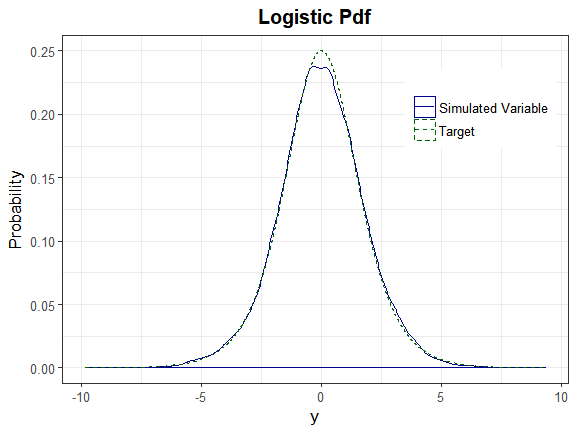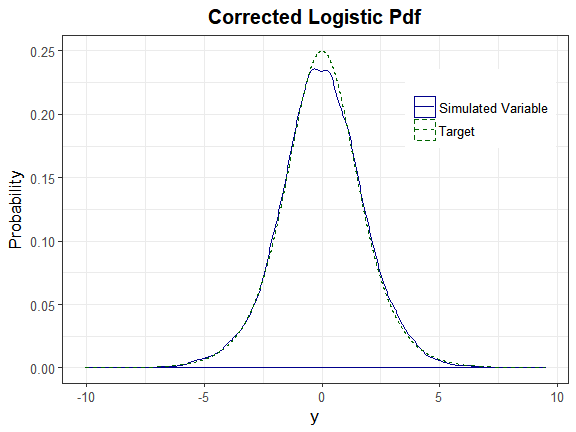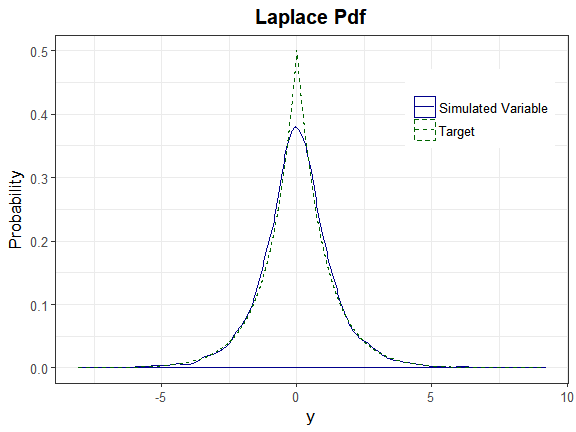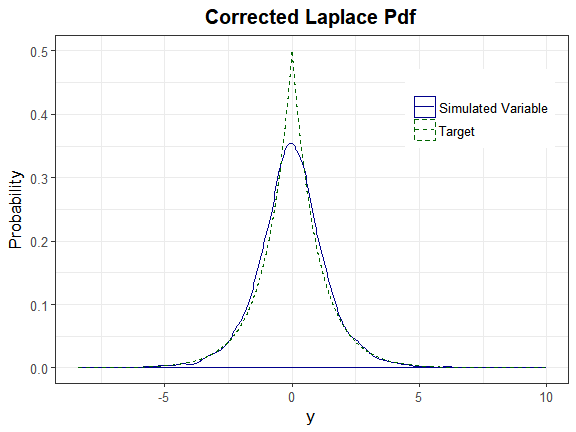# Using the Sixth Cumulant Correction to Find Valid Power Method Pdfs

#### 2018-06-28

Sometimes a target (continuous) distribution’s standardized cumulants yield polynomial transformation constants that generate an invalid power method pdf. This is an important consideration when utilizing a simulated distribution to perform power analysis or hypothesis testing. In the case of Headrick’s fifth-order approximation (2002), Headrick and Kowalchuk (2007) proposed that a correction can be used to increase the sixth cumulant’s value in order to produce a valid pdf. This is discussed in the help pages for find_constants and the simulation functions (nonnormvar1, rcorrvar, and rcorrvar2). These functions allow the user to input a Six vector of correction values, and then choose the smallest value that generates a valid pdf. When Headrick generated his Table 1 (2002, p.691 - 692) containing the fifth-order polynomial transformation constants for some common symmetrical and asymmetrical theoretical densities, he did not discuss whether the constants generate valid power method pdfs. His 2007 paper with Kowalchuk explained how to verify if a given set of constants generates a valid pdf (for both Fleishman’s third-order (1978) and Headrick’s fifth-order method). These checks are implemented in pdf_check, which is called by find_constants.

## Example

The following example illustrates the use of the sixth cumulant correction vector when finding the constants for each of Headrick’s Table 1 distributions (given in Headrick.dist), and compares distributions generated with the corrections to those generated without the corrections. (Note that the printr Xie (2017) package is invoked to display the tables.)

### Step 1) Find the theoretical standardized cumulants for each distribution.

The parameters are stored in H_params. The order of the columns corresponds to the order of the columns in Headrick.dist. The columns of H_params are named as inputs for calc_theory. Please see the appropriate R help page concerning the parameterization of each distribution. We are rounding the calculated standardized cumulants to 10 decimal places to ensure that the symmetric distributions have $$\Large \gamma_{1} = 0$$ and $$\Large \gamma_{3} = 0$$.

library("SimMultiCorrData")
library("printr")
H_stcum <- matrix(1, nrow = 4, ncol = ncol(Headrick.dist))
for (i in 1:ncol(H_params)) {
if (is.na(H_params[2, i])) {
params <- H_params[1, i]
} else {
params <- as.numeric(H_params[, i])
}
H_stcum[, i] <- round(calc_theory(Dist = colnames(H_params)[i],
params = params)[3:6], 10)
}
rownames(H_stcum) <- c("skew", "skurtosis", "fifth", "sixth")
round(H_stcum[, 1:6], 5)
Gaussian Logistic Uniform Laplace Triangular t_7df
skew 0 0.00000 0.00000 0 0.00000 0
skurtosis 0 1.20000 -1.20000 3 -0.60000 2
fifth 0 0.00000 0.00000 0 0.00000 0
sixth 0 6.85714 6.85714 30 1.71424 80
round(H_stcum[, 7:12], 5)
t_10df Chisq_1df Chisq_2df Chisq_3df Chisq_4df Chisq_8df
skew 0 2.82843 2 1.63299 1.41421 1.0
skurtosis 1 12.00000 6 4.00000 3.00000 1.5
fifth 0 67.88274 24 13.06394 8.48528 3.0
sixth 10 479.99841 120 53.33333 30.00000 7.5
round(H_stcum[, 13:18], 5)
Chisq_16df Chisq_32df Beta_a4b4 Beta_a4b2 Beta_a4b1.5 Beta_a4b1.25
skew 0.70711 0.50000 0.00000 -0.46771 -0.69388 -0.84815
skurtosis 0.75000 0.37500 -0.54545 -0.37500 -0.06860 0.22105
fifth 1.06066 0.37500 0.00000 1.40312 1.82825 1.90702
sixth 1.87500 0.46875 1.67832 -0.42614 -3.37911 -5.82705
round(H_stcum[, 19:22], 5)
Weibull_a6b10 Gamma_a10b10 Rayleigh_a0.5msqrt0.5pi Pareto_t10a1
skew -0.37326 0.63246 0.63111 2.81106
skurtosis 0.03546 0.60000 0.24509 14.82857
fifth 0.44706 0.75895 -0.31314 130.20815
sixth -1.02207 1.20000 -0.86829 1808.89959

Note that the standardized cumulants match those found by Headrick, except for the Gamma($$\Large \alpha = 10,\ \beta = 10$$) distribution. Either there is a mistake in Headrick’s table, or he is using a different parameterization.

### Step 2) Use the cumulants to find the constants for each distribution.

The sixth cumulant corrections will be chosen based on previous analysis in order to decrease computation time. If the user does not know what a sixth cumulant correction needs to be, a wide range with a small increment in values may be specified, i.e. Six = seq(0.1, 10, 0.1). In situations where the user has a better idea of the necessary correction, a smaller vector should be chosen. In the case of the Triangular distribution, the standardized kurtosis has been changed to the lower kurtosis boundary (found using calc_lower_skurt).

Six <- list(NULL, seq(1.7, 1.8, 0.01), seq(0.5, 2, 0.5), seq(25.1, 25.2, 0.01),
seq(0.1, 0.3, 0.01), NULL, NULL, seq(0.5, 2, 0.5),
NULL, NULL, NULL, NULL, NULL, NULL, NULL, NULL,
seq(0.01, 0.05, 0.01), seq(0.15, 0.2, 0.01), seq(0.5, 2, 0.5),
NULL, seq(0.5, 2, 0.5), seq(0.5, 2, 0.5))
H_consol <- list()

start.time <- Sys.time()
for (i in 1:ncol(H_stcum)) {
skurtsH <- ifelse(colnames(H_stcum)[i] == "Triangular", -0.5856216,
H_stcum[2, i])
H_consol[[i]] <- find_constants(method = "Polynomial", skews = H_stcum[1, i],
skurts = skurtsH, fifths = H_stcum[3, i],
sixths = H_stcum[4, i], Six = Six[[i]])
}
stop.time <- Sys.time()
Time <- round(difftime(stop.time, start.time, units = "min"), 3)
cat("Total computation time:", Time, "minutes \n")
## Total computation time: 0.019 minutes
H_cons <- matrix(1, nrow = 7, ncol = ncol(Headrick.dist))
for (i in 1:ncol(H_stcum)) {
H_cons[1:6, i] <- H_consol[[i]]$constants H_cons[7, i] <- ifelse(is.null(H_consol[[i]]$SixCorr1), NA,
H_consol[[i]]$SixCorr1) valid[i] <- H_consol[[i]]$valid
}
rownames(H_cons) <- c("c0", "c1", "c2", "c3", "c4", "c5", "sixcorr")

### Step 3) Look at results to see which distributions still have invalid power method pdfs

colnames(H_cons)[valid == FALSE]
##  "Uniform"                 "Chisq_1df"
##  "Weibull_a6b10"           "Rayleigh_a0.5msqrt0.5pi"
##  "Pareto_t10a1"

Therefore, the Uniform($$\Large 0,\ 1$$), Chisq($$\Large df = 1$$), Weibull($$\Large \alpha = 6,\ \beta = 10$$), Rayleigh($$\Large \alpha = 0.5,\ \mu = \sqrt{0.5 * \pi}$$), and Pareto($$\Large \theta = 10,\ \alpha = 1$$) distributions still have invalid power method pdf constants.

### Step 4) Look at constants and sixth cumulant corrections

round(H_cons[, 1:6], 6)
Gaussian Logistic Uniform Laplace Triangular t_7df
c0 0 0.000000 0.000000 0.000000 0.000000 0.000000
c1 1 0.887926 1.347438 0.782367 1.100185 0.907394
c2 0 0.000000 0.000000 0.000000 0.000000 0.000000
c3 0 0.036052 -0.140177 0.067899 -0.037538 0.014980
c4 0 0.000000 0.000000 0.000000 0.000000 0.000000
c5 0 0.000001 0.001808 0.000000 0.000632 0.002780
sixcorr NA 1.750000 2.000000 25.140000 0.160000 NA
round(H_cons[, 7:12], 6)
t_10df Chisq_1df Chisq_2df Chisq_3df Chisq_4df Chisq_8df
c0 0.000000 -0.397713 -0.307740 -0.259037 -0.227508 -0.163968
c1 0.920482 0.621096 0.800560 0.867102 0.900716 0.950794
c2 0.000000 0.416871 0.318764 0.265362 0.231610 0.165391
c3 0.021453 0.068429 0.033500 0.021276 0.015466 0.007345
c4 0.000000 -0.006386 -0.003675 -0.002108 -0.001367 -0.000474
c5 0.000830 0.000043 0.000159 0.000092 0.000055 0.000014
sixcorr NA 2.000000 NA NA NA NA
round(H_cons[, 13:18], 6)
Chisq_16df Chisq_32df Beta_a4b4 Beta_a4b2 Beta_a4b1.5 Beta_a4b1.25
c0 -0.116936 -0.083017 0.000000 0.108304 0.162966 0.200396
c1 0.975534 0.987806 1.093437 1.104253 1.089984 1.075051
c2 0.117433 0.083192 0.000000 -0.123347 -0.187329 -0.231504
c3 0.003573 0.001762 -0.035711 -0.045284 -0.044950 -0.044208
c4 -0.000166 -0.000058 0.000000 0.005014 0.008121 0.010369
c5 0.000004 0.000001 0.000752 0.001285 0.001445 0.001640
sixcorr NA NA NA NA 0.030000 0.180000
round(H_cons[, 19:22], 6)
Weibull_a6b10 Gamma_a10b10 Rayleigh_a0.5msqrt0.5pi Pareto_t10a1
c0 0.065524 -0.104760 -0.107593 -0.345859
c1 0.969217 0.980451 1.022480 0.712411
c2 -0.065172 0.105115 0.091123 0.346926
c3 0.027783 0.002843 -0.003307 0.028078
c4 -0.000117 -0.000118 0.005490 -0.000356
c5 -0.003879 0.000002 -0.002025 0.003962
sixcorr 2.000000 NA 2.000000 2.000000
1. The Gaussian, t($$\Large df = 10$$), Chisq($$\Large df = 2, ..., 32$$), Beta($$\Large \alpha = 4,\ \beta = 4$$), Beta($$\Large \alpha = 4,\ \beta = 2$$), and Gamma($$\Large \alpha = 10,\ \beta = 10$$) distributions had valid power method pdfs with the original cumulants.

2. The Logistic($$\Large 0, 1$$), Triangular($$\Large 0, 1$$), t($$\Large df = 7$$), Beta($$\Large \alpha = 4,\ \beta = 1.5$$), and Beta($$\Large \alpha = 4,\ \beta = 1.25$$) required relatively small sixth cumulant corrections.

3. The Laplace($$\Large 0, 1$$) required the largest correction at 25.14.

### Step 5) Simulate distributions

We will choose the Logistic($$\Large 0, 1$$) and Laplace($$\Large 0, 1$$) distributions for illustration.

First, simulate without the sixth cumulant correction.

seed <- 1234
Rey <- matrix(c(1, 0.4, 0.4, 1), 2, 2)

# Make sure Rey is within feasible correlation bounds
valid <- valid_corr(k_cont = 2, method = "Polynomial",
means = rep(0, 2), vars = rep(1, 2),
skews = H_stcum[1, c("Logistic", "Laplace")],
skurts = H_stcum[2, c("Logistic", "Laplace")],
fifths = H_stcum[3, c("Logistic", "Laplace")],
sixths = H_stcum[4, c("Logistic", "Laplace")],
rho = Rey, seed = seed)
##
##  Constants: Distribution  1
##
##  Constants: Distribution  2
## All correlations are in feasible range!
A <- rcorrvar(n = 10000, k_cont = 2, method = "Polynomial",
means = rep(0, 2), vars = rep(1, 2),
skews = H_stcum[1, c("Logistic", "Laplace")],
skurts = H_stcum[2, c("Logistic", "Laplace")],
fifths = H_stcum[3, c("Logistic", "Laplace")],
sixths = H_stcum[4, c("Logistic", "Laplace")],
rho = Rey, seed = seed)
##
##  Constants: Distribution  1
##
##  Constants: Distribution  2
##
## Constants calculation time: 0 minutes
## Intercorrelation calculation time: 0 minutes
## Error loop calculation time: 0 minutes
## Total Simulation time: 0 minutes

Look at the maximum correlation error:

cat(paste("The maximum correlation error is ", round(A$maxerr, 5), ".", sep = "")) ## The maximum correlation error is 0.00149. Look at the interquartile-range of correlation errors: Acorr_error = round(A$correlations - Rey, 6)
cat(paste("The IQR of correlation errors is [",
round(quantile(as.numeric(Acorr_error), 0.25), 5), ", ",
round(quantile(as.numeric(Acorr_error), 0.75), 5), "].", sep = ""))
## The IQR of correlation errors is [-0.00149, 0].

Second, simulate with the sixth cumulant correction.

Six <- list(H_cons["sixcorr", "Logistic"], H_cons["sixcorr", "Laplace"])

# Make sure Rey is within feasible correlation bounds
valid2 <- valid_corr(k_cont = 2, method = "Polynomial",
means = rep(0, 2), vars = rep(1, 2),
skews = H_stcum[1, c("Logistic", "Laplace")],
skurts = H_stcum[2, c("Logistic", "Laplace")],
fifths = H_stcum[3, c("Logistic", "Laplace")],
sixths = H_stcum[4, c("Logistic", "Laplace")],
Six = Six, rho = Rey, seed = seed)
##
##  Constants: Distribution  1
##
##  Constants: Distribution  2
## All correlations are in feasible range!
B <- rcorrvar(n = 10000, k_cont = 2, method = "Polynomial",
means = rep(0, 2), vars = rep(1, 2),
skews = H_stcum[1, c("Logistic", "Laplace")],
skurts = H_stcum[2, c("Logistic", "Laplace")],
fifths = H_stcum[3, c("Logistic", "Laplace")],
sixths = H_stcum[4, c("Logistic", "Laplace")], Six = Six,
rho = Rey, seed = seed)
##
##  Constants: Distribution  1
##
##  Constants: Distribution  2
##
## Constants calculation time: 0 minutes
## Intercorrelation calculation time: 0 minutes
## Error loop calculation time: 0 minutes
## Total Simulation time: 0.001 minutes

Look at the maximum correlation error:

cat(paste("The maximum correlation error is ", round(B$maxerr, 5), ".", sep = "")) ## The maximum correlation error is 0.00101. Look at the interquartile-range of correlation errors: Bcorr_error = round(B$correlations - Rey, 6)
cat(paste("The IQR of correlation errors is [",
round(quantile(as.numeric(Bcorr_error), 0.25), 5), ", ",
round(quantile(as.numeric(Bcorr_error), 0.75), 5), "].", sep = ""))
## The IQR of correlation errors is [-0.00101, 0].

In both cases, the correlation errors are small, indicating that the error loop does not need to be used.

Now compare the results numerically.

Target distributions:

as.matrix(round(A$summary_targetcont, 5), nrow = 2, ncol = 7, byrow = TRUE) Distribution mean sd skew skurtosis fifth sixth Logistic 1 0 1 0 1.2 0 6.85714 Laplace 2 0 1 0 3.0 0 30.00000 Without the sixth cumulant correction: as.matrix(round(A$summary_continuous[, c("Distribution", "mean", "sd", "skew",
"skurtosis", "fifth", "sixth")], 5), nrow = 2,
ncol = 7, byrow = TRUE)
Distribution mean sd skew skurtosis fifth sixth
X1 1 -0.00043 0.99694 -0.01936 1.03693 0.13868 5.22781
X2 2 0.00141 0.99907 0.06174 2.86625 1.36530 22.26331
A$valid.pdf ##  "FALSE" "FALSE" With the correction: as.matrix(round(B$summary_continuous[, c("Distribution", "mean", "sd", "skew",
"skurtosis", "fifth", "sixth")], 5), nrow = 2,
ncol = 7, byrow = TRUE)
Distribution mean sd skew skurtosis fifth sixth
X1 1 -0.00042 0.99694 -0.01820 1.02581 0.14315 6.01048
X2 2 0.00133 0.99847 0.06998 2.68915 1.91011 27.97101
B$valid.pdf ##  "TRUE" "TRUE" The distributions simulated with the sixth cumulant corrections are closer to the target distributions. Compare the results graphically. Logistic Distribution: plot_sim_pdf_theory(sim_y = A$continuous_variables[, 1],
title = "Logistic Pdf", Dist = "Logistic",
params = H_params$Logistic)plot_sim_pdf_theory(sim_y = B$continuous_variables[, 1],
title = "Corrected Logistic Pdf", Dist = "Logistic",
params = H_params$Logistic)Laplace Distribution: plot_sim_pdf_theory(sim_y = A$continuous_variables[, 2],
title = "Laplace Pdf", Dist = "Laplace",
params = H_params$Laplace)plot_sim_pdf_theory(sim_y = B$continuous_variables[, 2],
title = "Corrected Laplace Pdf", Dist = "Laplace",
params = H_params\$Laplace)